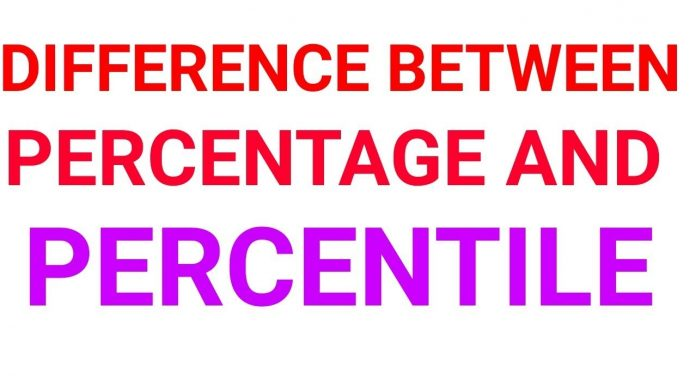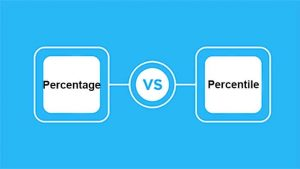A percentage is a mathematical term or number that is written out of a total of 100. The sign to indicate this term is percent “%,” and the denominator is 100. Percentages provide information about proportions or quantities and ratios. Difference between percentage and percentile, It is easy to use, comprehend and understand the differences when someone uses a percentage rather than fractions with different denominators.

## What is Percentage: Difference between percentage and percentile• Using a percentage serves as a most simple and easy way to standardize different quantities for evaluation purposes. It has many applications and can be applied in different circumstances, even in everyday life. For instance, if someone wishes to know what proportion of children in each school are males but there are a diverse number of students in each school. In this situation, it becomes compulsory to convert the numbers to percentages and then compare the two schools. It will be easy to see if one school has a more significant proportion of male students than the other school by standardizing.
• This is possible to write Percentages as ratios and decimals, so 50% is the same as 5/10 and 0.50.
• It is common practice to use Percentages for the calculation of interest rates in the financial world as well as calculating the grades of students at school or college.
• Percentages can also used to indicate changes in values, such as upturns or reductions. For example, a student’s grade may increase from one test to another, so maybe he has improved by 10%. This is very valuable as it keeps the potential to show change over time of some value.
• A percentage is a mathematical quantity that is not used much as a statistical quantity, although some statistical tests can analyze percentage data.

## What is Percentile

• A percentile is a percentage of all those values which can found below a specific value. In this way, it is related to percentage. For instance, if the achieved 75th percentile on a test is 160, it means that if a student scored 160, he scored better than 75% of the people who took that same test.
• A percentage can’t change the value, so the 75% will always be 75/100. In contrast, a percentile can change.
• Percentiles are applicable in standardized tests to create a ranking system of accomplishment. It  constructes by dividing up a normal distribution of values into percentiles.  The most important are the 25th, 50th, and 75th percentile.
• Percentage and percentile do not essentially signify the same quantity. For instance, in the case of SAT, the score of 60% on the test may give you the 40th percentile. It is that it depends on how many other people scored 60% out of the total number. On the other hand, the percentage will be the individual score. Percentile can use in scoring standardized tests as well as in statistics.
• Percentiles keep quartiles while percentages have no quartiles system.  We can write Percentages in decimals, but this is not the case with percentiles.

Also read: how often can you donate blood